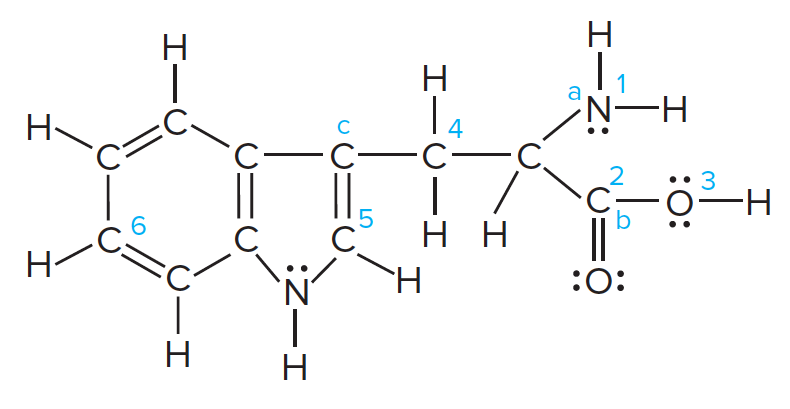Chemistry Practice Problems Bond Angles Practice Problems Solution: Tryptophan is one of the amino acids found in prot...

⚠️Our tutors found the solution shown to be helpful for the problem you're searching for. We don't have the exact solution yet.

# Solution: Tryptophan is one of the amino acids found in proteins:Predict the bond angles at points a, b, and c.

###### Problem

Tryptophan is one of the amino acids found in proteins:Predict the bond angles at points a, b, and c.

Bond Angles

Bond Angles

#### Q. Name the shape and give the AXmEn classification and ideal bond angle(s) for each of the following general molecules:

Solved • Sun Oct 28 2018 04:22:58 GMT-0400 (EDT)

Bond Angles

#### Q. Why is the H–N–H angle in NH3 smaller than the H–C–H bond angle in CH4? Why is the H–N–H angle in NH4+ identical to the H–C–H bond angle in CH4?

Solved • Mon Oct 22 2018 15:27:30 GMT-0400 (EDT)

Bond Angles

#### Q. Compare the shapes and bond angles of these oxynitrogen ions. NO3-, NO2-, NO43-, NO2+

Solved • Wed Apr 04 2018 10:24:47 GMT-0400 (EDT)

Bond Angles

#### Q. Determine the electron geometry, molecular geometry, and idealized bond angles for each of the following molecules. In which cases do you expect devia...

Solved • Fri Mar 30 2018 10:41:46 GMT-0400 (EDT)